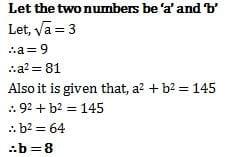Test: Squares And Square Roots- 1

# Test: Squares And Square Roots- 1

Test Description

## 10 Questions MCQ Test Mathematics (Maths) Class 8 | Test: Squares And Square Roots- 1

Test: Squares And Square Roots- 1 for Quant 2023 is part of Mathematics (Maths) Class 8 preparation. The Test: Squares And Square Roots- 1 questions and answers have been prepared according to the Quant exam syllabus.The Test: Squares And Square Roots- 1 MCQs are made for Quant 2023 Exam. Find important definitions, questions, notes, meanings, examples, exercises, MCQs and online tests for Test: Squares And Square Roots- 1 below.
Solutions of Test: Squares And Square Roots- 1 questions in English are available as part of our Mathematics (Maths) Class 8 for Quant & Test: Squares And Square Roots- 1 solutions in Hindi for Mathematics (Maths) Class 8 course. Download more important topics, notes, lectures and mock test series for Quant Exam by signing up for free. Attempt Test: Squares And Square Roots- 1 | 10 questions in 10 minutes | Mock test for Quant preparation | Free important questions MCQ to study Mathematics (Maths) Class 8 for Quant Exam | Download free PDF with solutions
 1 Crore+ students have signed up on EduRev. Have you?
Test: Squares And Square Roots- 1 - Question 1

### Which of the following is a perfect square number?

Detailed Solution for Test: Squares And Square Roots- 1 - Question 1

The answer is 1681 because it is the only number which has it's last digit as a number which a perfect square can have . 9×9=81 the last digit is 1.

Test: Squares And Square Roots- 1 - Question 2

### Which of the following would end with digit 1?

Detailed Solution for Test: Squares And Square Roots- 1 - Question 2

Option B is correct because the unit digit of 161 is 1 and if unit digit of any digit ends with 1 the its square will also end with 1.

Test: Squares And Square Roots- 1 - Question 3

### Without adding, find the sum. 1 + 3 + 5 + 7 + 9

Detailed Solution for Test: Squares And Square Roots- 1 - Question 3

Here, we have to find the sum of first five odd natural numbers.

Therefore, 1 + 3 + 5 + 7 + 9 = (5)2 = 25

Test: Squares And Square Roots- 1 - Question 4

Find the smallest whole number by which 1008 should be multiplied to get a perfect square number .

Detailed Solution for Test: Squares And Square Roots- 1 - Question 4

The smallest whole number is 7, by which 1008 should be multiplied so as to get a perfect square.

Now 1008×7= 7056

=2×2×2×2×3×3×7×7

So, the square root will be =2×2×3×7 = 84

Test: Squares And Square Roots- 1 - Question 5

When a square number ends in _______, the number whose square it is, will have either 4 or 6 in unit’s place.

Detailed Solution for Test: Squares And Square Roots- 1 - Question 5

Answer is 6 because the last digit of the square of 4 is 6 and 4 is also 6.

Test: Squares And Square Roots- 1 - Question 6

Sum of squares of two numbers is 145. If square root of one number is 3, find the other number.

Detailed Solution for Test: Squares And Square Roots- 1 - Question 6Test: Squares And Square Roots- 1 - Question 7

Find the perfect square numbers between 30 and 40.

Detailed Solution for Test: Squares And Square Roots- 1 - Question 7

Since, 1 x 1 = 1

2 x 2 = 4

3 x 3 = 9

4 x 4 = 16

5 x 5 = 25

6 x 6 = 36

7 x 7 = 49

Thus, 36 is a perfact square number between 30 and 40.

Test: Squares And Square Roots- 1 - Question 8

All square numbers end with __________ at unit’s place.

Detailed Solution for Test: Squares And Square Roots- 1 - Question 8

The numbers ending with 0 gives 0,numbers ending with 1,9 gives 1, numbers ending with 2,8 gives 4, numbers ending with 3,7 gives 9, numbers ending with 5 gives 5 and numbers ending with 4,6 gives 6. Hence we get only 0,1,4,5,6,9 at the unit's place.

Test: Squares And Square Roots- 1 - Question 9

Without doing any calculation, find the numbers which are surely perfect squares.

Detailed Solution for Test: Squares And Square Roots- 1 - Question 9

The perfect squares have 0,1,2,4,5,6 or 9 at their units place.
Therefore153,257 and 408 are surely not perfect squares.

Test: Squares And Square Roots- 1 - Question 10

How many numbers lie between square of 12 and 13

Detailed Solution for Test: Squares And Square Roots- 1 - Question 10

122 = 12*12 = 144

132 = 13*13 = 169

Now numbers are between144 and 169 are:

145, 146, 147,.............168

Total number = 24

So total numbers lies between 144 and 169 is 24

## Mathematics (Maths) Class 8

193 videos|360 docs|48 tests
 Use Code STAYHOME200 and get INR 200 additional OFF Use Coupon Code
Information about Test: Squares And Square Roots- 1 Page
In this test you can find the Exam questions for Test: Squares And Square Roots- 1 solved & explained in the simplest way possible. Besides giving Questions and answers for Test: Squares And Square Roots- 1, EduRev gives you an ample number of Online tests for practice

## Mathematics (Maths) Class 8

193 videos|360 docs|48 tests